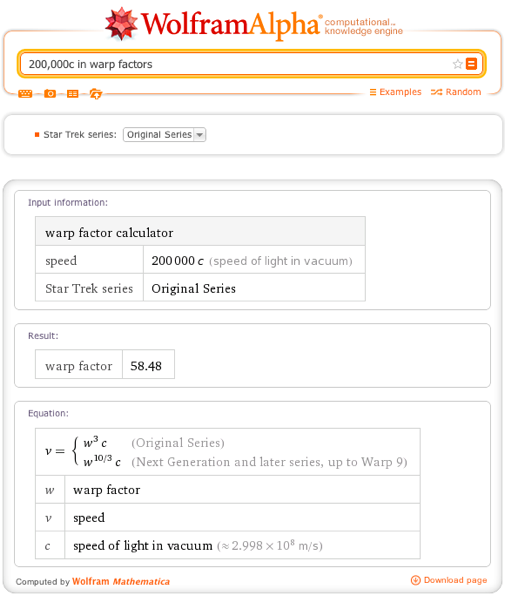# “Helm, Warp One, Engage!” Calculating Warp Speed Factors

July 23, 2012 —Comments Off

Wolfram|Alpha’s goal is to cover all things computational, from mathematics and the sciences to movies and sports. But the set of all things computable encompasses areas outside of the real world as well. With the 25th anniversary of Star Trek: The Next Generation coming up, we can now compute the relationship between warp factors and the speed of light.

Warp factors describe the speed for a spaceship, such as the Starship Enterprise, traveling faster than the speed of light within the Star Trek universe. In the real world, even approaching the speed of light is outside of our current capabilities. To get the sense of energies involved, we can start with the mass of the space shuttle. This comparatively small spaceship turns out to weigh about 81,000 kilograms. The kinetic energy of 81,000 kilograms at 0.99999c is 1.621*10^24 joules. This in turn is about 42 times the world’s estimated fossil fuel reserves as of 2003. So, for now, even fast subluminal velocities are outside of our reach.

But in Star Trek, faster than light travel is a well developed technology. However, exact conversion of warp factor into multiples of the speed of light is somewhat complicated by the fact that the writers often neglected the established formulas in their scripts and that there are two versions of the formula used over the course of the various Star Trek series. The first formula was introduced in the original series’ writer’s guide by Gene Roddenberry, and was used for the original series. This the default formula used by Wolfram|Alpha when asked about warp factors such as warp factor 3.An interesting aspect of this formula is that unlike in the latter series, there is no restriction against warp factors above 10 in the original series, a fact that came up in several episodes. If we ask about 200,000c in warp factors, we see that this would be equivalent to a warp factor of over 58 in the original series.In Star Trek: The Next Generation and series that followed it, a limit was placed on the maximum warp factor possible (even if the writers again often ignored this restriction). In this revised warp factor framework, we have a new formula that covers warp factors up to 9. Thus we can ask about Warp Factor 7 in Deep Space 9 or Voyager at Warp Factor 5.

Above warp 9, speed is supposed to increase exponentially to infinity as one approaches warp 10. There was never a hard formula given for this, but we have tried to approximate it by interpolating over the data extracted from the hand-drawn curve used by the production team for The Next Generation series. So we can still give an approximate answer to the question of warp factor 9.5 in Next Generation.A log plot of the interpolated data shows the speed rapidly heading to infinity as one approaches warp factor 10.Reverse queries are also possible with this model. We might ask what warp factor is needed to cross the Milky Way. The diameter of the Milky Way is about 100,000 light years, which is similar to the distance the characters in the series Star Trek: Voyager needed to cross to return home. In that series it was estimated that it would take them 75 years to complete by normal means. That works out to an average speed of 100,000 light years / 75 years or 1334c. 1334c in warp factors for Voyager works out to be warp factor 8.661.

We hope that you enjoy using this formula and discovering the speeds that James Tiberius Kirk or Jean-Luc Picard traveled at while boldly going where no man had gone before.

Would be interesting to see the relative time calculation. So how long it ‘appears’ to take the Enterprise to travel these distances from a viewer on Earth 🙂

Posted by Jim Shuker July 23, 2012 at 4:59 pm

A nice present for the Trekkie fans. So to get to Alpha Centauri in one day, using Voyager’s warp factors, I would need a warp factor of around 9.14. This is so cool.

Posted by Dom Calisto July 26, 2012 at 6:35 am

@Jim I’m pretty sure relativity doesn’t apply in subspace.

Two problems I have with this function:
1. It tops out at Warp 9.999. Any faster and it falls back to 2154 c. (Warp 9.998 is 30578 c.)
2. It’s “off” compared to other calculators. For Warp 9.999, the calculator I use gets 10267 c. Wolfram|Alpha gives 48839 c.

 http://blog.shields-online.net/?page_id=65 << Martin Shields is one of the guys who /really/ tried to determine the WF formula as seen here: http://www.calormen.com/Star_Trek/FAQs/warp_velocities-faq.htm

Posted by Tesla Ranger August 20, 2012 at 12:18 pm

Voyager had a path of 75000ly.

Posted by Jovan Miloskovski August 11, 2013 at 1:38 am